Precalculus : Use Product/Sum Identities to Express a Sum or Difference as a Product

Example Questions

Example Question #221 : Pre Calculus

Evaluate the following.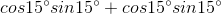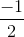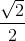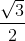Explanation:

We can use the angle sum formula for sine here.

If we recall that,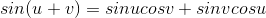,

we can see that the equation presented is equal to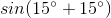because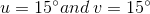.

We can simplify this to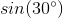, which is simplyExample Question #2 : Product/Sum Identities

Evaluate the following.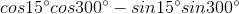Explanation:

The angle sum formula for cosine is,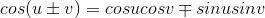.

First, we see that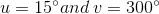. We can then rewrite the expression as,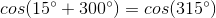All that is left to do is to recall the unit circle to evaluate,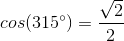.

Example Question #1 : Use Product/Sum Identities To Express A Sum Or Difference As A Product

Evaluate the following.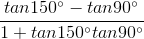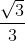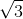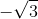Explanation:

This one is another angle sum/difference problem, except it is using the trickier tangent identity.

The angle sum formula for tangent is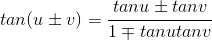We can see that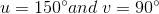.

We can then rewrite the expression as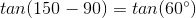, which is.

Example Question #1 : Product/Sum Identities

Evaluate the following.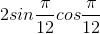Explanation:

Here we use the double angle identity for sine, which is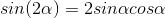We can rewrite the originial expression as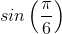using the double angle identity.

From here we can calculate that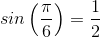.

Example Question #5 : Product/Sum Identities

Evaluate the following expression.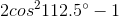Explanation:

One of the double angle formuals for cosine is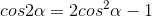We can use this double angle formula for cosine to rewrite the expression given as the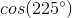because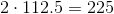and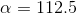.

We can then calculate that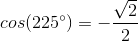.

Example Question #1 : Product/Sum Identities

Evaluate the following.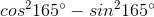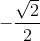Explanation:

Here we can use another double angle formula for cosine,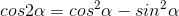.

Here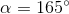, and so we can use the double angle formula for cosine to rewrite the expression as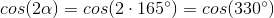.

From here we just recognize that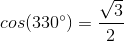.

Example Question #231 : Pre Calculus

Evaluate the following expression.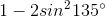Explanation:

Here we can use yet another double angle formula for cosine: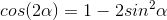.

First, realize that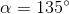.

Next, plug this in to the double angle formula to find that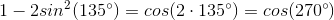.

Here we recognize that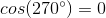Example Question #1 : Product/Sum Identities

Simplify the following. Leave your answer in terms of a trigonometric function.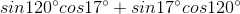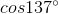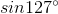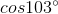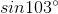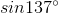Explanation:

This is a quick test of being able to recall the angle sum formula for sine.

Since,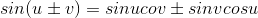, and here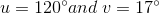, we can rewrite the expression as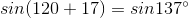.

All Precalculus Resources